Homework Help Question & Answers

# Research the melting point, boiling point, heat of fusion, heat of vaporization, and heat capacity values...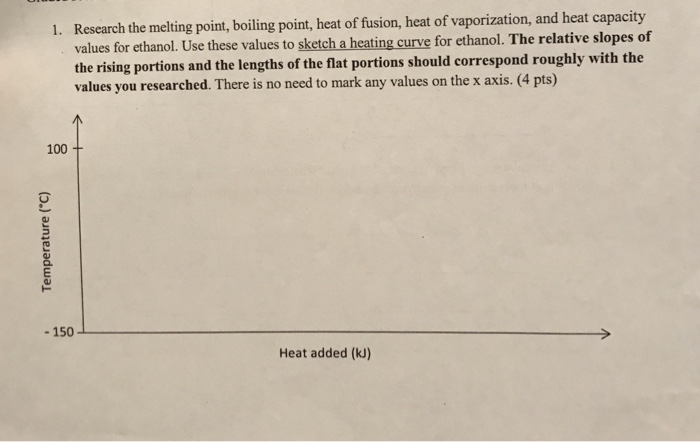Research the melting point, boiling point, heat of fusion, heat of vaporization, and heat capacity values for ethanol. Use these values to sketch a heating curve for ethanol. The relative slopes of the rising portions and the lengths of the flat portions should correspond roughly with the values you researched. There is no need to mark any values on the x axis. (4 pts) 1. 100+ -150 Heat added (kJ)

#### Homework Answers

Answer #1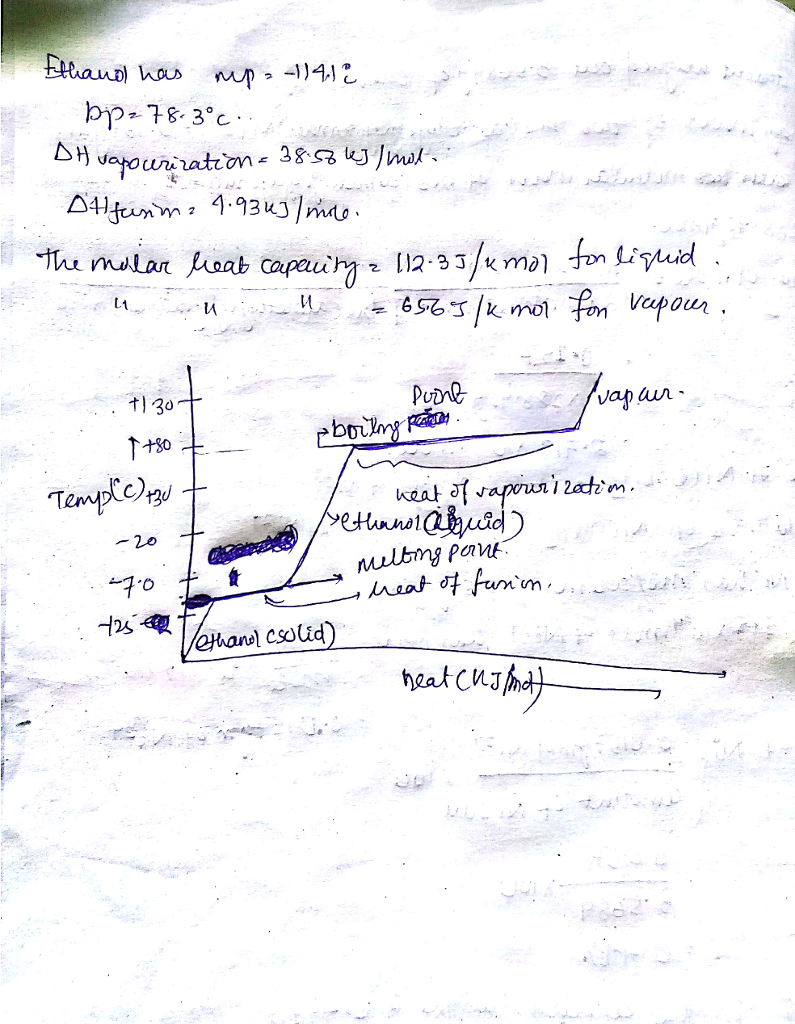Know the answer?
Your Answer:

#### Post as a guest

Your Name:

What's your source?

#### Earn Coin

Coins can be redeemed for fabulous gifts.

Not the answer you're looking for? Ask your own homework help question. Our experts will answer your question WITHIN MINUTES for Free.
Similar Homework Help Questions
• ### How much heat energy is required to convert 15.0 g of solid ethanol at -114.5 °C...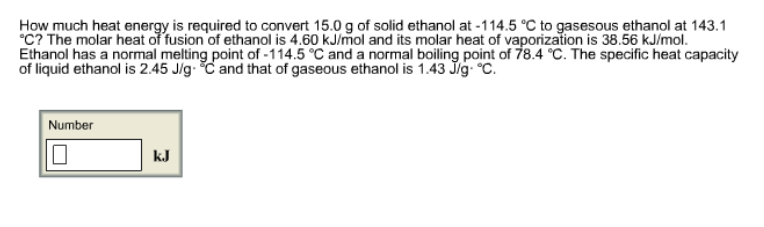How much heat energy is required to convert 15.0 g of solid ethanol at -114.5 °C to gasesous ethanol at 143.1 °C? The molar heat of fusion of ethanol is 4.60 kJ/mol and its molar heat of vaporization is 38.56 kJ/mol. Ethanol has a normal melting point of -114.5 °C and a normal boiling point of 78.4 °C. The specific heat capacity of liquid ethanol is 2.45 J/g·°C and that of gaseous ethanol is 1.43 J/g·°C. How much heat energy...

• ### potassium metal has melting point of 63.65 C and a boiling point of 774 C Enthalpy...

potassium metal has melting point of 63.65 C and a boiling point of 774 C Enthalpy fusion = 14.37 cal/g enthaply of vaporization = 490.3 cal/g Specific heat @ solid = .1759 cal/gC specfic heat @ liquid =.5425 cal /gC specfic heat @ gas = .2629 cal /gC a) draw heatung curve that us heated from 23C to 1200c b) Calculate total heat required in units of kJ for 39.5 g of potassium

• ### How much heat energy is required to convert 19.8 g of solid ethanol at –114.5 °C...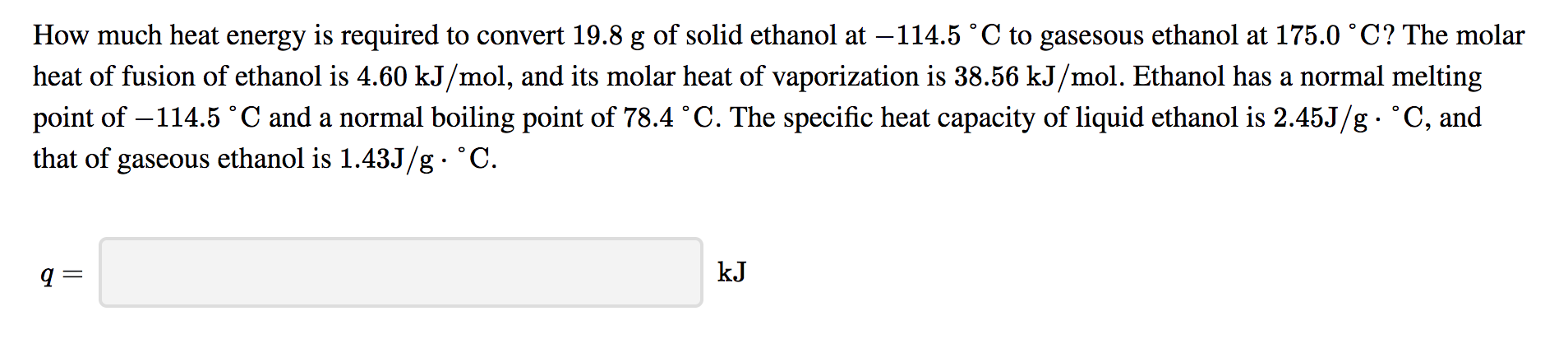How much heat energy is required to convert 19.8 g of solid ethanol at –114.5 °C to gasesous ethanol at 175.0 °C? The molar heat of fusion of ethanol is 4.60 kJ/mol, and its molar heat of vaporization is 38.56 kJ/mol. Ethanol has a normal melting point of –114.5 °C and a normal boiling point of 78.4 °C. The specific heat capacity of liquid ethanol is 2.45J/g • °C, and that of gaseous ethanol is 1.43J/g. °C.

• ### How much heat energy is required to convert 49.7 g of solid ethanol at –114.5 °C...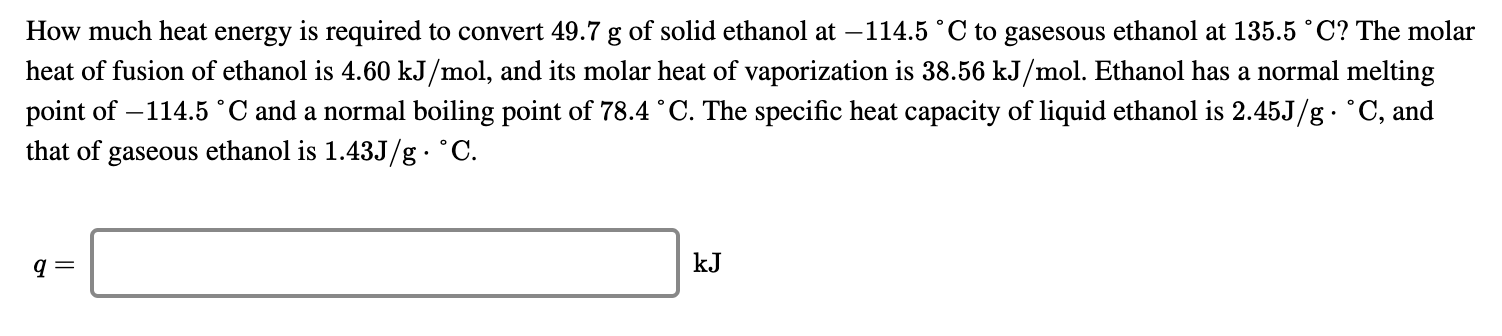How much heat energy is required to convert 49.7 g of solid ethanol at –114.5 °C to gasesous ethanol at 135.5 °C? The molar heat of fusion of ethanol is 4.60 kJ/mol, and its molar heat of vaporization is 38.56 kJ/mol. Ethanol has a normal melting point of -114.5 °C and a normal boiling point of 78.4 °C. The specific heat capacity of liquid ethanol is 2.45J/g. °C, and that of gaseous ethanol is 1.43J/g • °C. kᎫ

• ### How much heat energy is required to convert 16.2 g of solid ethanol at -114.5 °C...

How much heat energy is required to convert 16.2 g of solid ethanol at -114.5 °C to gasesous ethanol at 191.9 °C? The molar heat of fusion of ethanol is 4.60 kJ/mol and its molar heat of vaporization is 38.56 kJ/mol. Ethanol has a normal melting point of -114.5 °C and a normal boiling point of 78.4 °C. The specific heat capacity of liquid ethanol is 2.45 J/g·°C and that of gaseous ethanol is 1.43 J/g·°C.

• ### For ethanol, C2H5OH, the heat of fusion at its normal melting point of -115 °C is...

For ethanol, C2H5OH, the heat of fusion at its normal melting point of -115 °C is 5.0 kJ/mol. The entropy change when 1.84 moles of solid C2H5OH melts at -115 °C, 1 atm is J/K.

• ### calculate the heat required to melt 9.73 g of benzene at it's normal melting point nd...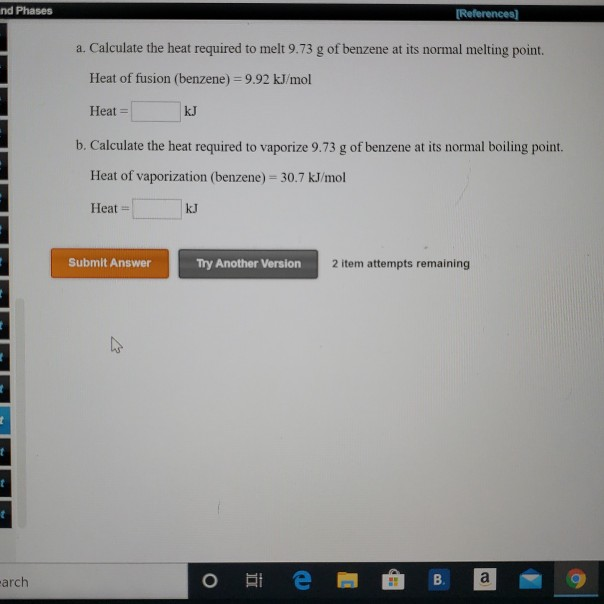calculate the heat required to melt 9.73 g of benzene at it's normal melting point nd Phases (References] a. Calculate the heat required to melt 9.73 g of benzene at its normal melting point. Heat of fusion (benzene) 9.92 kJ/mol Heat = kJ b. Calculate the heat required to vaporize 9.73 g of benzene at its normal boiling point. Heat of vaporization (benzene) = 30.7 kJ/mol Heat = kJ Submit Answer Try Another Version 2 item attempts remaining arch о...

• ### Resources Hint Check Answer Question 8 of 10 > How much heat energy is required to...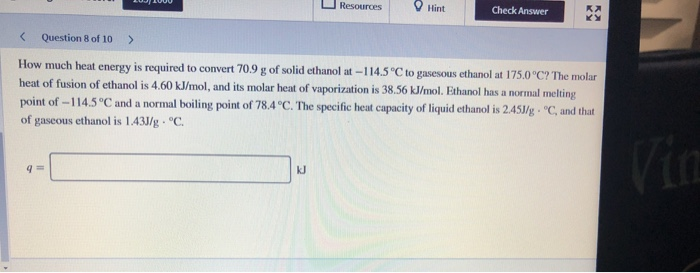Resources Hint Check Answer Question 8 of 10 > How much heat energy is required to convert 70.9 g of solid ethanol at-114.5 °C to gasesous ethanol at 175.0°C? The molar heat of fusion of ethanol is 4.60 kJ/mol, and its molar heat of vaporization is 38.56 kJ/mol. Ethanol has a normal melting point of -114.5°C and a normal boiling point of 78.4°C. The specific heat capacity of liquid ethanol is 2.453/g • °C, and that of gaseous ethanol is...

• ### just #6 Thermochemistry, Part I, Calorimetry, Homework 7 Specific Heat Values: cal; Diamond(0.124), graphite(0.170), ice(0.50), steam(0.48),...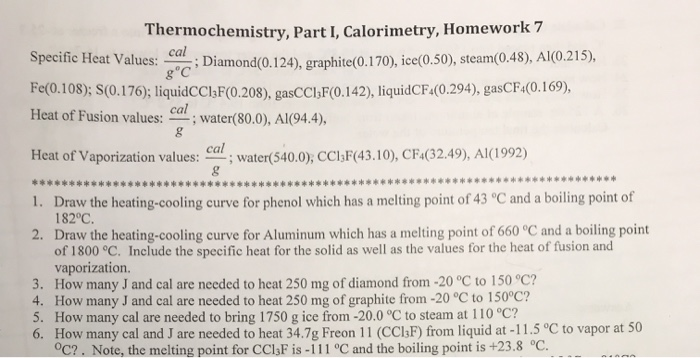just #6 Thermochemistry, Part I, Calorimetry, Homework 7 Specific Heat Values: cal; Diamond(0.124), graphite(0.170), ice(0.50), steam(0.48), Al(0.215), Fe(0.108); S(0.176); liquidCCIF(0.208), gasCCI;F(0.142), liquidCF4(0.294), gasCF4(0.169), Heat of Fusion values: cal; water(80.0), Al(94.4), Heat of Vaporization values: Car; water(540.0), CC13F(43.10), CF4(32.49), Al(1992) 1. Draw the heating-cooling curve for phenol which has a melting point of 43 ºC and a boiling point of 182°C. 2. Draw the heating-cooling curve for Aluminum which has a melting point of 660 °C and a boiling point of...

• ### Using the following heating curve, what line represents the melting point ["AB", "DE", "CD", "BC", "EF"] ? What line rep...

Using the following heating curve, what line represents the melting point ["AB", "DE", "CD", "BC", "EF"] ? What line represents the boiling point ["AB", "CD", "EF", "DE", "BC"] ? Where would you use the specific heat capacity of a solid  ["DE", "EF", "AB", "BC", "CD"] ? Specific heat capacity of a liquid  ["DE", "AB", "CD", "EF", "BC"] ? Specific heat capacity of a solid ["AB", "CD", "DE", "EF", "BC"] ? Where would you use the heat of fusion (ΔHfus) ["EF", "AB", "DE",...